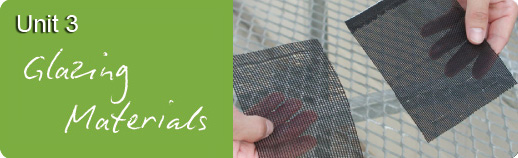Printer Friendly VersionSection 4: Other Considerations

Sometimes, such as in subtropical and tropical locations, heating is not required, and the amount of light entering the greenhouse is above optimal levels. Additionally, high temperatures may be an important concern. In these cases, a shading material made from polypropylene or some type of fabric may be used as the glazing instead of a solid transparent glazing (such as those discussed above). The shading material reduces the amount of light entering the structure and also reduces ambient temperature (it also potentially provides protection from wind). Various shading materials that reduce light transmittance by 10% to 90% are available. Some of these materials such as saran are usually black and the amount of light excluded depends of the thickness of the material's weave. Other types of shading glazings are made from fabric with various amounts and dimensions of thin aluminum strips or fibers incorporated that actually reflect light away from the structure.

Maintenance of Greenhouse Glazings
Greenhouse glazings should periodically be washed on the inside and the outside to remove dirt and debris that block light (reduces transmittance) and scratches the glazing. Glazings that have reached their maximum life span and begun to yellow should be replaced. The glazing and mounting should be inspected before heating season to insure that the glazing is mounted properly without gaps between panels which can allow for significant heat loss from the greenhouse.

Calculating Greenhouse Surface Area
It is often necessary to calculate surface area of a greenhouse in order to determine the amount of glazing required (for example if polyethylene film is being replaced). To calculate surface areas, a greenhouse manager only needs to know several basic geometric equations.

Circumference of a circle = 2πr or πd
Area of a circle = πr2
Total surface area of a cylinder = (2π rH) + (2π r2)
Area of a right (90°) triangle = 0.5 x (LH)
Area of a rectangle (parallelogram) = L x W
Volume of a cube = L x W x H
Sides of a right triangle determined by a2 + b2 = c2
Where π = 3.14, r is the radius, H is the height, L is the length and W is the width. Further a is the length of one leg of a right triangle, b is the other leg and c is the hypotenuse (long side).

Using these basic equations we can calculate the surface area of two example structures.

The first structure is a quonset house without extended side walls. The structure is 50 feet in length and 20 feet wide. Calculations can be determined by assuming the structure is essentially a cylinder cut in half (therefore the front and back surfaces are half circles).

Surface area:

0.5 x [(2π rH) + (2π r2)]
0.5 x [(2 x 3.14 x 10 ft x 50 ft) + (2 x 3.14 x 10 ft2)]
0.5 x [(3140 ft2) + (628 ft2)]
=1884 ft2

The second structure is an A-frame or a free-standing gable greenhouse. The structure is 30 feet wide, 6 feet tall (side walls) and 100 feet long. The roof section is also 100 feet long and each roof section is 20 feet wide. The structure can be broken down into cubes, rectangles and triangles.

Surface Area:

-- Top Part:
(roof) L x W
(20 ft x 100 ft) x 2 = 4000 ft2

The gable is a little more difficult to calculate. Because we do not know if the top angle is a right triangle we can't multiply the sides (20 x 20) and must we split the gable into two parts that we know are right triangles by drawing a line from the peak down to the top of the wall portions (splitting the gable in half). This gives us a right triangle with the bottom leg being 15 feet, the hypotenuse being 20 feet and the upright leg is unknown in its length. We can calculate the unknown leg using a2+b2=c2 (Pythagorean Theorem!).

With this we have:
a2+152=202 (all in feet)
a2+225=400 ft2
a2=175 ft2
a=13.2 ft2

So we have a right triangle (half of the gable) that has one leg (bottom) of 15 feet and the other (upright) is 13.2 feet. The area of the right triangle is 0.5 (13.2 x 15) = 99 ft2. We have 4 of the right triangles (two per gable). So the total gable surface area is 396 ft2.

Top surface area = 4000 ft2 + 396 ft2 = 4396 ft2

-- Bottom Part:
L x W
(30 ft x 6 ft) x 2 = 360 ft2
(100 ft x 6 ft) x 2 = 1200 ft2
Bottom surface area = 360 ft2 + 1200 ft2 = 1560 ft2

Total Surface Area = 4396 ft2 + 1560 ft2 = 5956 ft2

You are now at the end of Unit 3: Glazing Materials. Click here to take the self-exam.
Your test results will be sent to Dr. Evans.